# Hole

They fill shape hole with dimensions 2.9 m, 17 m, 15.2 m with 97 m3 of soil. How many percent it fill up?

Correct result:

p =  13 %

#### Solution:

$p = 100 \cdot \dfrac{ 97 } { 2.9\cdot 17\cdot 15.2} = 13 \%$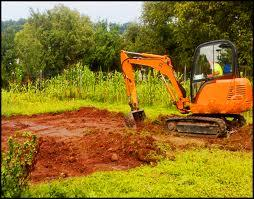We would be very happy if you find an error in the example, spelling mistakes, or inaccuracies, and please send it to us. We thank you!Tips to related online calculators

## Next similar math problems:

• Living roomHow many people can live in a room with dimensions: a = 4m b = 5m c = 2.5m if one person needs 15m cubic space (i. E. Air . .. )?
• A store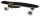A store received a shipment of 240 skateboards. In three weeks, it sold 1/3 of those skateboards. How many skateboards did the store sell?
• Fractions 4How many 2/3s are in 6?
• Farmers 2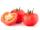On Wednesday the farmers at the Grant Farm picked 2 barrels of tomatoes. Thursday, the farmers picked 1/2 as many tomatoes as on Wednesday. How many barrels of tomatoes did the farmers pick on Thursday?
• The resultHow many times I decrease the number 1632 to get the result 24?
• Scouts 4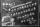4/7 of the students in a school are boys. If 3/8 of the boys are scouts, how many scouts are there in a school of 1878 students?
• Math classificationIn 3A class are 27 students. One-third got a B in math and the rest got A. How many students received a B in math?
• One third 2One third of all students in class live in a house. If here are 42 students in a class, how many of them live in house?
• 100 %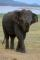How much is 100%, if 17 % is 1169?
• Pie II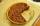Vili ate three pieces of pie. If it pieces is 1/8 how much pie did he eat?
• Double 5Peter was thinking of a number. Peter doubles it and gets an answer of 8.6. What was the original number?
• Two divided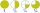Two divided by nine tenths.
• PieVili ate three pieces of pie . If it piece is 1/8 how much pie did he eat?
• Pizza 5You have 2/4 of a pizza and you want to share it equally between 2 people how much pizza does each person get?
• Product of two fractionsProduct of two fractions is 9 3/5 . If one of the fraction is 9 3/7. Find the other fraction.
• Date game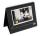Let now is Wednesday. What day is after 248 days? Write the result as a number: 1=Monday, 2=Tuesday, 3=Wednesday, 4=Thursday, 5=Friday, 6=Saturday, 7=Sunday?
• One-third 2One-third of the people in a barangay petitioned the council to allow them to plant in vacant lots and another 1/5 of the people petitioned to have a regular garbage collection. What FRACTION of the barangay population made the petition?